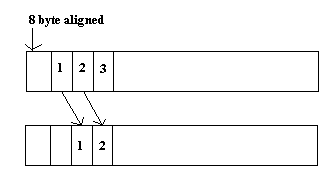# TI DSPLIB Modifications

When I wrote this page, I didn't know yet that I wouldn't be able to include the modified source. I'm allowed to distribute the original. But if I make any changes, I can only distribute object files or executables. So, I've removed the modified code. You'll just have to make do with the descriptions and the object files.

While working on my thesis, I modified a couple of TI's DSPLIB optimized routines. The first one was the cache optimized mixed radix IFFT routine. I modified it so that the output is divided by four. This was done so that I could do two FFTs and IFFTs simultaneously by making use of the imaginary part of the time domain signal. It runs in the exact same number of cycles as the original.

The second routine I modified was the dot product function. I'm going to go over the original and then talk about my changes. First notice that the prototype requires a pointer to the first array, then a pointer to the second, and then the length of the arrays. The arrays must be double word aligned. Next take a look at the assignments. The array length goes into A_cntarg (A6). The first array is A_x (A4), and the second is B_x (B4). A_x0 and A_x1 are adjacent entries in the array. The same goes for B_x0 and B_x1. The loop has been unrolled so that at each pass, prod0 is the even product and prod1 is the odd product. They are stored as a running sum in sum0 and sum1.

The remaining cycles of the epilog sum the partial sums in several stages. The final result is put into A_result. The branch occurs five cycles before the end of the routine. It jumps back to the address stored in B3.

For my needs, the data cannot be aligned on eight bytes. I modified the routine so that the first element of the first array starts at four bytes shifted from an eight byte alignment. The second array is eight byte aligned. The number of elements must be a multiple of four. Or the end of the arrays can be padded with zeros.The loop is unrolled once more so I can get an extra instruction in. This is necessary because the arrays are no longer aligned in memory. I have to temporarily store the second element loaded from the first array so that it can be used in the next iteration of the loop.

The first cycle of the loop performs the branch. It also moves the second element, A_x1, into A_tmp. The next cycle decrements count by four. It also again puts A_x1 in A_tmp. This will be used on the next cycle to multiply the first element of B, B_x0. And A_x0 will be multiplied by B_x1.

The prolog is similar to the original. But branches only happen every other instruction. And cnt isn't decremented on the last prolog cycle (or the loop would never end). The epilog drops one additional add cycle because this happens inside the loop. Also, the odd logic has been removed because I require the input array size to be a multiple of four.

Here are the object and header files. You call them just like the originals.
DSPF_sp_dotprod2.obj
DSPF_sp_dotprod2.h
DSPF_sp_ifftSPxSPscale.obj
DSPF_sp_ifftSPxSPscale.h

And the original source.
DSPF_sp_dotprod.h
DSPF_sp_dotprod.asm
DSPF_sp_ifftSPxSP.asm

home